# Count Total Cells in a Table – Excel & Google Sheets

In this tutorial, we will learn how to convert a table into a range, count the table’s rows, columns, and the total cells in the table.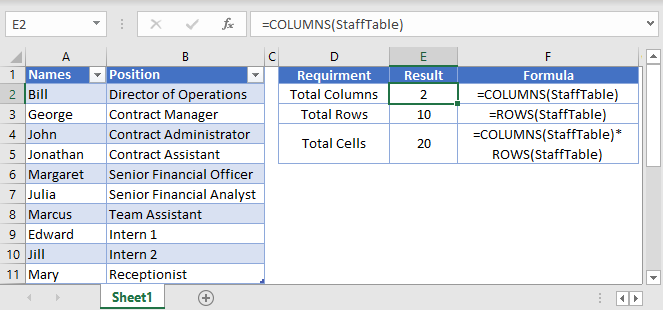## Check Table Name

First you’ll want to check and/or update the Table Name for use in the formula. Here we will name our table StaffTable.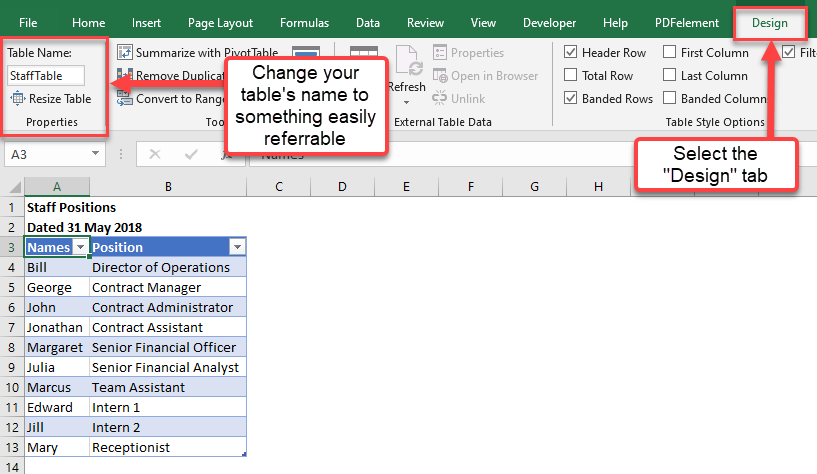## Count Table Rows and Table Columns

``=ROWS(Table Name) - NOTE: Excludes Table Headers``
``=COLUMNS(Table Name)``

We will use the ROWS function to count the table’s rows (the ROWS function will not count the table headers):

``=ROWS(StaffTable2)``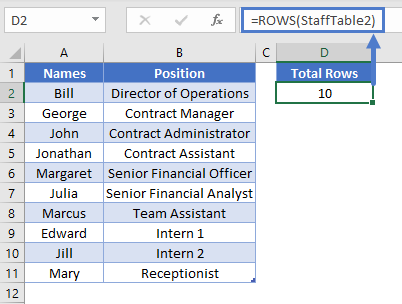We will use the COLUMNS Function in the same way:

``=COLUMNS(StaffTable24)``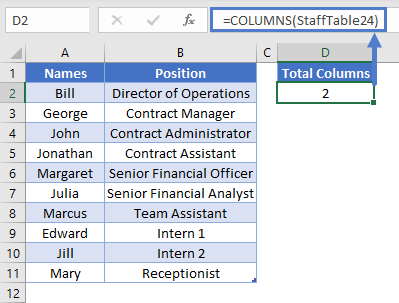## Count Total Cells in a Table

``=ROWS(Table Name)*COLUMNS(Table Name)``

We can use the ROWS function and the COLUMNS function to find the total number of cells. By multiplying the total number of rows with the total number of columns in the same table, this gives us the total number of cells.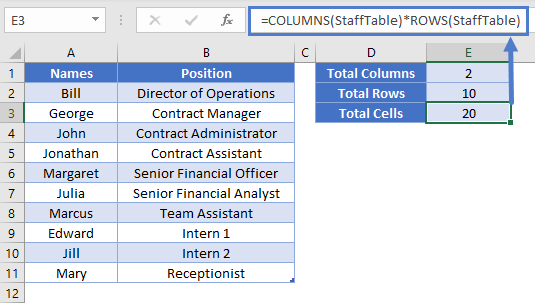The end result is 20 cells.  As advised earlier, please note that it is not counting the table’s headers in the calculation of the total rows.

## Google Sheets – Count Total Cells in a Table

All of the above examples work exactly the same in Google Sheets as in Excel.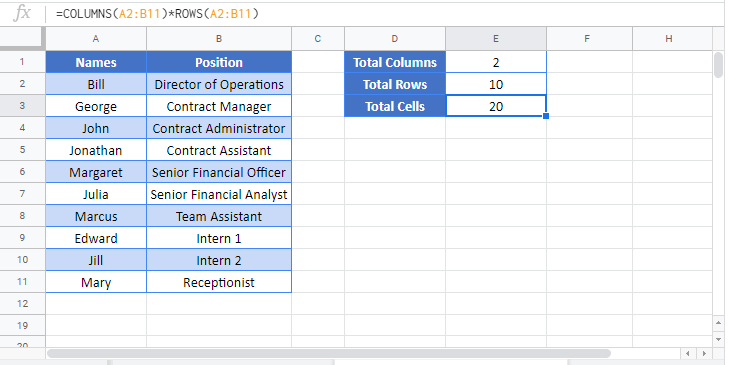### Excel Practice Worksheet

Practice Excel functions and formulas with our 100% free practice worksheets!